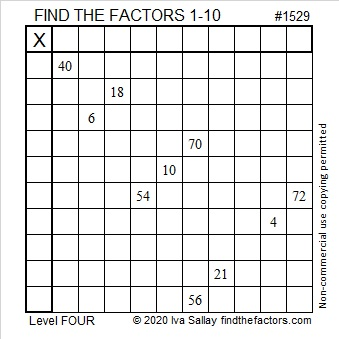# 1529 Pointy Hat

Contents

#### Today’s Puzzle:

A pointy hat is part of many different Halloween costumes: Wizards, Witches, Medieval Princesses, and Clowns come to my mind. Today’s puzzle looks like a pointy hat. Use logic to work your magic in solving it! As always, there is only one solution.### Factors of 1529:

Look at this math fact using the digits of 1529:
1 – 5 + 2 – 9 = -11, a number divisible by 11, so 1529 is divisible by 11.

• 1529 is a composite number.
• Prime factorization: 1529 = 11 × 139.
• 1529 has no exponents greater than 1 in its prime factorization, so √1529 cannot be simplified.
• The exponents in the prime factorization are 1 and 1. Adding one to each exponent and multiplying we get (1 + 1)(1 + 1) = 2 × 2 = 4. Therefore 1529 has exactly 4 factors.
• The factors of 1529 are outlined with their factor pair partners in the graphic below.### More about the Number 1529:

1529 is the difference of two squares in two different ways:
765² – 764²  = 1529, and
75² – 64²  = 1529.

This site uses Akismet to reduce spam. Learn how your comment data is processed.